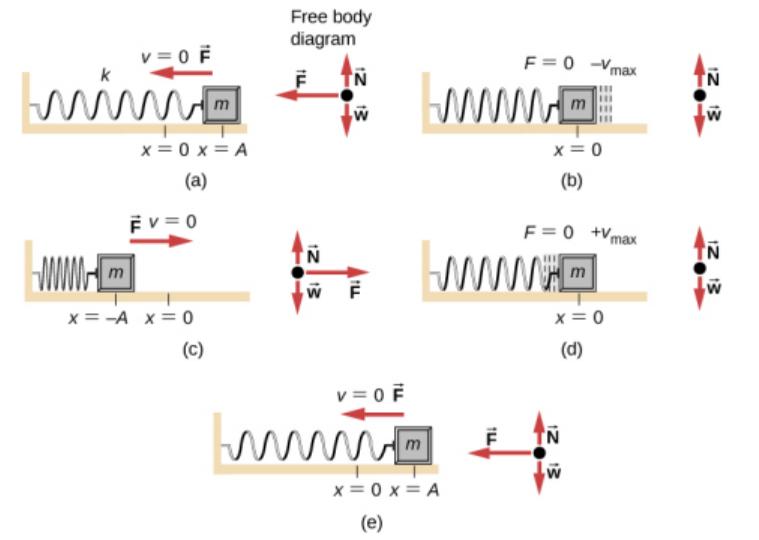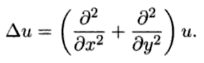# Harmonic Function / Motion (Potential Function)

A harmonic function (called a potential function in physics) is a real-valued function with continuous second partial derivatives that satisfy the Laplace equation. The equation is satisfied when Δu = 0, where Δ is the Laplace operator.

The definition isn’t very intuitive to grasp, but it’s based on simple harmonic motion that up and down motion of a spring, which can be represented by sine functions and cosine functions. In multivariable calculus (i.e. in higher dimensions), that type of motion happens on the unit n-sphere, and is represented by the Laplace equation. That’s simplifying the definition a lot, but the definition itself isn’t meant to be enlightening; the harmonic function definition is just an equation—one that’s meant to be relatively easy to work with (Davis, 2016).

## Simple Harmonic Motion

Simple harmonic motion is a special kind of repetitive back and forth movement. In simple harmonic motion, the movement is through a central position called the equilibrium, and the maximum displacement on one side of the equilibrium is the same as the maximum displacement on the other side.

The secret behind this type of motion is the force: the force which creates the motion is directed toward the equilibrium position and is always directly proportional to the distance from it. What this means is that the further we go from the equilibrium, the stronger the force that pulls us toward it.

## Example of Simple Harmonic Motion

A basic example of simple harmonic motion is the way a spring, connected to a weight, would vibrate on a friction-less surface after being displaced by your hand.• Image a shows the spring when you have just pulled the object a distance of X and then released. The instantaneous velocity is 0, but the spring is exerting a force on the spring in an effort to get back to x = 0.
• In image b, the weight has reached the equilibrium and force is zero, but the velocity is at the highest it will be and it will carry the weight onward to the left.

The further the weight moves to the left, the greater the force to the right becomes. Eventually it cancels out the velocity, and the weight is sent back to the right, where it crosses the equilibrium with the same maximum velocity it just crossed it in the other direction. Since we have a friction-less system, the weight will return to the exact same place we pulled it to originally.

We call the time it takes to make a complete cycle the period T. The bigger our weight, the greater the time T. Also, the stiffer the spring, the smaller the time T.

## Harmonic Function Examples

Harmonic functions are found in a wide variety of settings as diverse as animation, electric circuits, heat distribution and random walks. In physics, harmonic functions are determined by their singularities and boundary conditions (conditions that have to be satisfied at the boundary of a region where you’re solving a set of differential equations).

The real and imaginary parts of any holomorphic function are two-variable harmonic functions.

## Formal Definition of a Harmonic Function

The formal definition of a harmonic function is (Greene & Krantz, 2006):

A real-valued function u: U → ℝ on an open set ∪ ⊆ ℂ is harmonic if it is C2 on U and Δu ≡0, where the operator Δu is Laplacian, defined as:If you’re unfamiliar with mathematical/set notation:

## References

Ash, J. M. (Ed.). Studies in Harmonic Analysis. Washington, DC: Math. Assoc. Amer., 1976.
Benedetto, J. J. Harmonic Analysis and Applications. Boca Raton, FL: CRC Press, 1996.
Cohn, H. Conformal Mapping on Riemann Surfaces. New York: Dover, 1980.
Davis, S. (2016). Voltage, Temperature, and Harmonic Functions. Retrieved January 10, 2020 from: Voltage, Temperature, and Harmonic Functions
Green, R. & Krantz, S. (2006). Function Theory of One Complex Variable. American Mathematical Society.
Krantz, S. G. “Harmonic Functions.” §1.4.1 and Ch. 7 in Handbook of Complex Variables. Boston, MA: Birkhäuser, pp. 16 and 89-101, 1999.